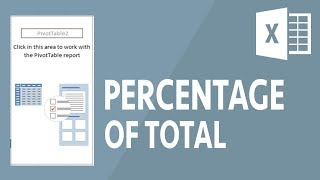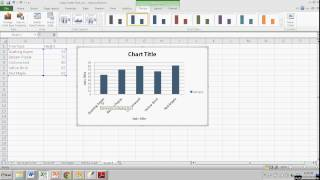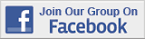Excel Tips & Tutorials:
Descriptive Statistics
 Suggest a Link Alphabetize Page Printer-Friendly List
Find data. Produce results. Tell the world.
Watch and Learn
Watch and Learn
Watch and Learn
Watch and Learn
 How to Show % of Total in PivotTableHelps create percentages column in frequency distribution table (4:30).How to Make a Map ChartDemonstrates using Florida zip codes (4:33).How to Use PivotTables to Summarize DataNice introduction and demonstration (17:39).Making a Simple Bar GraphScott Stevens creates the graph from scratch (5:13)How to Show % of Total in PivotTableHelps create percentages column in frequency distribution table (4:30).How to Make a Map ChartDemonstrates using Florida zip codes (4:33).How to Use PivotTables to Summarize DataNice introduction and demonstration (17:39).How to Show % of Total in PivotTableHelps create percentages column in frequency distribution table (4:30).How to Make a Map ChartDemonstrates using Florida zip codes (4:33).How to Show % of Total in PivotTableHelps create percentages column in frequency distribution table (4:30).Over 18,000 Members!